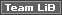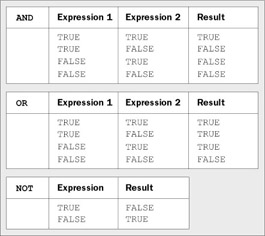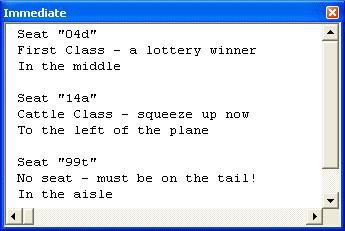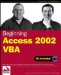# Programming StructuresThere are three main structures that are used in programming. Firstly, sequential , where we need to do things one after the other, or in a certain order. We saw some of that in the previous chapter, and you'll see plenty more as we go through the book. Next comes selection , where we often need to make a choice based upon some piece of information, so we either do one thing or another. Lastly comes looping, or repetition , where we do the same thing over and over again.

### Expressions

With VBA, you can check something and perform different operations depending on the results of the check. This check is usually called a condition or an expression . An expression always has a Boolean result - that is, it's either True or False . The easy way to think about expressions is to just think about everyday decisions, like 'Do I want to open a large tub of ice cream?' That's probably going to be True (it is for me, anyway). You are not limited to just one statement - how about 'Do I want to stop work and open a large tub of ice cream?' Here, we've got two statements.

When dealing with multiple statements you generally join them together, either by an And or by an Or . In the above example we used And , which means that both statements have to be True for the whole expression to be True . If you want to stop work but don't feel like ice cream at the moment, then the expression is False . If we had used Or , then only one of the statements needed to have been True for the whole expression to be True . 'Do I want to stop work Or do I want to open a large tub of ice cream?'

When joining statements together in expressions, the rule is quite simple:

• If you use Or to join the statements, then only one statement has to be True for the expression to be True .

• If you use And to join the statements, then all statements have to be True for the expression to be True .

You can also mix the two types of statements. 'Do I want to stop work and open a large tub of ice cream, or do I want a cup of coffee?'

You'll see plenty of expressions as we go through this chapter, and we'll be revisiting them again in a little while, once we've looked at how we make decisions.

### Decision Structures

We know that everyday life has many decisions, and VBA code often has plenty of them too. We use decision structures to make those decisions; these structures are the VBA statements that mean we can perform one task or another.

#### If...Then...

We've already come across this statement back when we were creating an intelligent navigation button. We'll now take a look at the structure of the statement and then run through a couple of examples. You use If ... Then when you want to execute a piece of code only when a certain statement is true. It has two formats; the single line format is as follows :

`   If expression Then statement   `

The multi-line format runs like this:

`   If expression Then     statement1     statement2     End If   `

Both of the above perform the same operation. (The second example, however, allows more than one line as the statement ). For example:

`   If strName = "" Then Exit Function   `

is the same as:

`   If strName = "" Then     Exit Function     End If   `

Here, you test whether the argument strName is an empty string (denoted by the two double quotes together), and if so, exit the function directly.

The expression can also contain other functions:

`   Sub Test()     Dim strNumber As String     strNumber = "1234"     If IsNumeric(strNumber) Then     Debug.Print strNumber & " is a number"     End If     strNumber = "one two three four"     If IsNumeric(strNumber) Then     Debug.Print strNumber & " is a number"     End If     End Sub   `

The function IsNumeric returns a value of True if the string passed in is a number. The above code would print out the following:

` 1234 is a number `

There would be nothing printed for the second example If statement because the string passed in does not contain a number.

### If...Then...Else...

You can use the If ... Then ... Else ... statement to decide which of two actions to perform. Let's revisit our number test, adding in a few lines:

` Sub Test()   Dim strNumber As String   strNumber = "1234"   If IsNumeric(strNumber) Then     Debug.Print strNumber & " is a number"   Else     Debug.Print strNumber & " is not a number"   End If   strNumber = "one two three four"   If IsNumeric(strNumber) Then     Debug.Print strNumber & " is a number"   Else     Debug.Print strNumber & " is not a number"   End If End Sub `

Here we test the string to see if it contains a number. If it does, we run the code as before. If the string is not a number, then we run some other code. This would produce:

` 1234 is a number one two three four is not a number `

You can include variables and numerical and relational operators in your expressions too:

`   Dim intAbc As Integer     Dim intDef As Integer     Dim strName As String     intAbc = 1     intDef = 2     strName = "Janine"     If intAbc = 1 Then Debug.Print "Abc is 1"     If intAbc = intDef Then Debug.Print "Two variables the same"     If intAbc > intDef Then Debug.Print "Abc is greater than Def"     If intAbc + 1 = intDef Then Debug.Print "Adding 1 to Abc gives Def"     if strName = "Janine" Then Debug.Print "Hello Jan"   `

Note that you can use any normal arithmetic operators within an If statement, such as +, -, *, /, or the relational operators < , > , = in conjunction with variables and values, to determine whether or not a condition is true.

### ElseIf...

The ElseIf statement is used for joining a set of If conditions together. This is quite common when you need to check the results of several different conditions:

`   If intAbc = 1 Then     Debug.Print "Abc is 1"     ElseIf intAbc = intDef Then     Debug.Print "Two variables the same"     Else     Debug.Print "Abc is not 1 and it is not the same as Def"     End If   `

If the first condition is true, then only the code between the Then statement and the ElseIf is executed and no more conditions are tested . If the first condition isn't true, the second is tried. If that isn't true, the Else statement is executed.

### Logical Operators with the If statement

You can also make more complex queries with this statement by using logical operators. The three most common logical operators are And , Or and Not . You can use these to test a combination of expressions together to get a true or false answer. The answer is calculated via a set of truth tables which are applied for each operator:It's as easy, though, to use common sense to deduce what the answer should be. Think of it in terms of the English language; for example, if you break the speed limit OR you rob a bank, it's true that you've broken the law. Let's look at the earlier example to make the criteria slightly more complex.

` Dim intAbc As Integer Dim intDef As Integer Dim strName As String intAbc = 1 intDef = 2 strName = "Janine"   If intAbc = 1 And intDef = 2 Then Debug.Print "Abc is 1 and Def is 2"     If intAbc = 1 Or intDef = 2 Then Debug.Print "Either Abc is 1 or Def is 2"   `

### Select Case

There's no limit to the number of ElseIf statements that you can have:

`   If datOrderDate >= #7/1/02# Then   ...   ElseIf datOrderDate >= #6/1/02# And datOrderDate <= #6/30/02# Then   ...   ElseIf datOrderDate < #6/1/02# Then   ...   Else   ...   End If   `

Remember that the # sign around the dates just tells VBA that this is a date value, otherwise VBA would take 6/1/02 as 6 divided by 1 divided by 2.

You can see that the code is starting to look messy. There's a much better way - using the Select Case statement:

`   Select Case datOrderDate     Case Is >= #7/1/02#     MsgBox "Your order was placed during or after July, 2002"     Case #6/1/02# To #6/30/02#     MsgBox "Thank you for your June, 2002 order."     ...     Case Else     ...     End Select   `

This is much clearer to read. If datOrderDate is equal to or after the 1 st of July then the section of code under the first Case statement would be executed. The second Case statement checks for datOrderDate being from June 1 st to June 30 th , and any code within the Case Else will be run if datOrderDate is any other date. Adding a Case Else statement is not compulsory, but it's always a good idea to include one, just in case the variable you are testing has an unexpected value. Even if you don't think you need a Case Else , it's best to put one in anyway, and put an error message there. That way, if some unexpected value appears you'll know about it, and be able to act accordingly .

You can also test for more than one value with Case :

`   Select Case intMainCount     Case 1, 2, 3   ...   Case 4 To 6   ...   Case Else   ...   End Select   `

This shows two different ways of testing the condition. If intMainCount is 1 , 2 , or 3 , the first section is executed. If it's between 4 and 6 inclusive, the second section is executed, and so on. You can achieve the same result using the Is keyword and an expression:

`   Select Case intMainCount     Case Is < 4   ...   Case Is < 7   ...   Case Else   ...   End Select   `

Here, if intMainCount is less than 4 , the first section is executed, and so on.

Note that, as soon as a true expression is found in a Select Case statement, no more expressions are checked. An expression must fail one test to get to the next. This means that if you have an expression that matches two Case statements, only the first will be executed.

Select Case isn't limited to numeric tests - you can also use strings:

`   Select Case strSalutation     Case "Mrs", "Miss", "Ms"   ...   Case "Mr"   ...   Case Else   ... End Select `

You can also use the To form with strings:

`   Case "Alfred" To "Bertrand"   `

This would be executed if the condition matched any string within the range specified. Don't be put off by the fact the string has a range - strings are checked in alphabetical order, so Alfred comes before Bertrand . They can be tested alphabetically , so a value such as Basil would be accepted in such a condition, while Roy would be excluded.

As you can see, not only is the Select Case statement very flexible, but it can also greatly increase the clarity of your code. Let's give this a go:

### Try It Out-Select Case

1. Create a new module, or if you are using the complete database go into the Chapter 04 Code module.

2. Create a new procedure called Seat , to find out where we are sitting on a plane. Add the following code:

`   Public Sub Seat(ByVal strSeatNumber As String)     Dim intRow As Integer     Dim strSeat As String     intRow = CInt(Left(strSeatNumber, 2))     strSeat = Right(strSeatNumber, 1)     Select Case intRow     Case 1     Debug.Print "At the front eh? Must be the pilot"     Case 2 To 5     Debug.Print "First Class - a lottery winner"     Case 6 To 10     Debug.Print "Business Class - on a business trip"     Case 11 To 54     Debug.Print "Cattle Class - squeeze up now"     Case Else     Debug.Print "No seat - must be on the tail!"     End Select     Select Case strSeat     Case "a" To "c"     Debug.Print "To the left of the plane"     Case "d" To "g"     Debug.Print "In the middle"     Case "h" To "j"     Debug.Print "To the right of the plane"     Case Else     Debug.Print "In the aisle"     End Select     End Sub   `
3. Switch to the Immediate window and try it out with a few seat numbers . These should have a two-digit row and a single character seat.How It Works

Firstly we have two variables, to store the row number and the seat letter.

`   Dim intRow As Integer     Dim strSeat As String   `

Before we can decide where you are sitting, we need to work out the row number. We use Left for this, which extracts the leftmost characters from a string. Here, we are extracting the two characters at the left of the strings, as these will be the row number. We assign this to the integer variable, allowing VBA to automatically convert the string containing numbers into an integer number.

`   intRow = Left(strSeatNumber, 2)   `

We then use Right for a similar purpose, this time to extract the very rightmost character from the string. This will be the seat number.

`   strSeat = Right(strSeatNumber, 1)   `

Now we can go ahead with our Select statement, first checking the row number. The first case will only happen if the row number is 1:

`   Select Case intRow     Case 1     Debug.Print "At the front eh? Must be the pilot"   `

If the row is between 2 and 5 inclusive then the second case is run:

`   Case 2 To 5     Debug.Print "First Class - a lottery winner"   `

For seats including 6 to 10 we have the third case:

`   Case 6 To 10     Debug.Print "Business Class - on a business trip"   `

For the seats at the back of the plane, 11 through to 54, the fourth case is run:

`   Case 11 To 54     Debug.Print "Cattle Class - squeeze up now"   `

If your row number is anything else then I hope you brought a coat, as it's quite windy on the tail!

`   Case Else     Debug.Print "No seat - must be on the tail!"     End Select   `

Once the row number is decided, you can find out which side of the plane you are on. Seats a to c are on the left, d to g are in the middle, and h to j are on the right.

`   Select Case strSeat     Case "a" To "c"     Debug.Print "To the left of the plane"     Case "d" To "g"     Debug.Print "In the middle"     Case "h" To "j"     Debug.Print "To the right of the plane"     Case Else     Debug.Print "In the aisle"     End Select   `

You can easily see how much clearer this is than if we had used If statements.

### IIf

There are certain places (such as in queries, but more on that later) where you need to be able to return one of two values, but you can't use the If statement. In these cases, you can use the immediate if , or IIf :

`   strName = "Janine"     strWhoAreYou = IIf (strName = "Janine", "Hi Jan", "Who are you?")   `

The IIf statement takes three arguments:

• The condition to test for

• The value to return if the condition was True

• The value to return if the condition was False

So, the statement below is exactly the same as the If statement above:

`   If strName = "Janine" Then     strWhoAreYou = "Hi Jan"     Else     strWhoAreYou = "Who are you?"     End If   `

Some people prefer using the IIf since it looks slightly neater, as it's all on one line, but it can be a cause of confusion, especially for new programmers. There is also a major drawback you have to be aware of. When using the IIf statement, all three arguments are evaluated by VBA. "So what?" you may ask. Consider the following examples, where we divide one number by another:

`   Public Function Divide (ByVal intNumber1 As Integer, ByVal intNumber2 As Integer) As Double     If intNumber2 = 0 Then     Divide = 0     Else     Divide = intNumber1 / intNumber2     End If     End Function     Public Function Divide (ByVal intNumber1 As Integer, ByVal intNumber2 As Integer) As Double     Divide = IIf (intNumber2 = 0, 0, intNumber1 / intNumber2)     End Function   `

The two functions look as though they should work the same, but this isn't the case. If intNumber2 is , the second version will give a Divide by Zero error because intNumber1 / intNumber2 is always evaluated. You must bear this in mind when you use IIf .

Because IIf evaluates all the arguments, it's slower to use than the normal If statement. Admittedly, you'll probably never notice this, but if you were to use IIf in a large loop, that small delay would gradually build up. You might not think that a small delay is a problem, but you can be sure that your users will think otherwise!

The real use for IIf is in queries and on forms and reports . You'll see examples of this later in the book.

### Operator Precedence

When combining conditions and expressions, it's very important that you understand operator precedence. This defines the order in which parts of an expression are evaluated and is similar to the lessons that you learnt in mathematics when you were at school. The rules are recapped below and may, at first, seem complex, but do persevere. There are some examples later to make everything clear.

When operators from more than one category are combined in an expression, arithmetic operators are evaluated first, comparison operators next, and logical operators last. The order of operator evaluation is shown below, from top downwards:

Arithmetic

Arithmetic Symbol

Exponentiation

^

Negation

-

Multiplication and Division

* /

Integer division

\

Modulo arithmetic

Mod

+ -

String concatenation

&

Some miscellaneous points are:

• All comparison operators, such as = , <, and Like , have equal precedence, the same level as addition and subtraction. This means that they are evaluated from left to right as they appear in expressions.

• Arithmetic operators with the same precedence are also evaluated from left to right.

• Operations within parentheses (brackets, like this) are always performed before those outside. This means that you can force the order in which evaluation takes place by using parentheses. However, normal precedence is maintained within the parentheses.

If that all sounds rather complex, don't worry. Here are some examples to help you.

You have four numbers: A , B , C, and D , and you want to multiply the sum of A and B by the sum of C and D :

`   A = 1     B = 2     C = 3     D = 4     E = A + B * C + D   `

This doesn't produce 21 , but 11 , as multiplication has a higher precedence than addition. What happens is that B and C are multiplied, then A and D are added. To correct this, use:

`   E = (A + B) * (C + D)   `

This forces the additions to be performed first. In the example below, the parentheses have no effect (although, to some, they make the intention clearer):

`   E = A * B + C * D     E = (A * B) + (C * D)   `

With expressions in If statements, you have to follow a similar set of rules for using And and Or . You can liken And to * and Or to + in the previous examples, since And has a higher order of precedence. For example, consider the following, where A, B, C, and D are all integers, all with the value of 1 :

`   If A = 1 Or B = 1 And C = 1 Or D = 1 Then   `

This expression will be True if any of these conditions are True :

• A is equal to 1

• B is equal to 1 and C is equal to 1

• D is equal to 1

However, consider the expression if we add parentheses:

`   If (A = 1 Or B = 1) And (C = 1 Or D = 1) Then   `

This expression will be True only if these conditions are True :

• Either A is equal to 1 or B is equal to 1

• Either C is equal to 1 or D is equal to 1

This is a fairly simplistic example, but you can clearly see the differences between the two sets of expressions. Most of the time, you will find that your expressions are more advanced, but it's important to know what happens when things get more complicated.

If you are at all unsure of the order of precedence of an expression, use parentheses to force your meaning. If the order was correct anyway, then you won't have lost anything, plus you will have made your code clearer.Beginning Access 2002 VBA (Programmer to Programmer)
ISBN: 0764544020
EAN: 2147483647
Year: 2003
Pages: 256